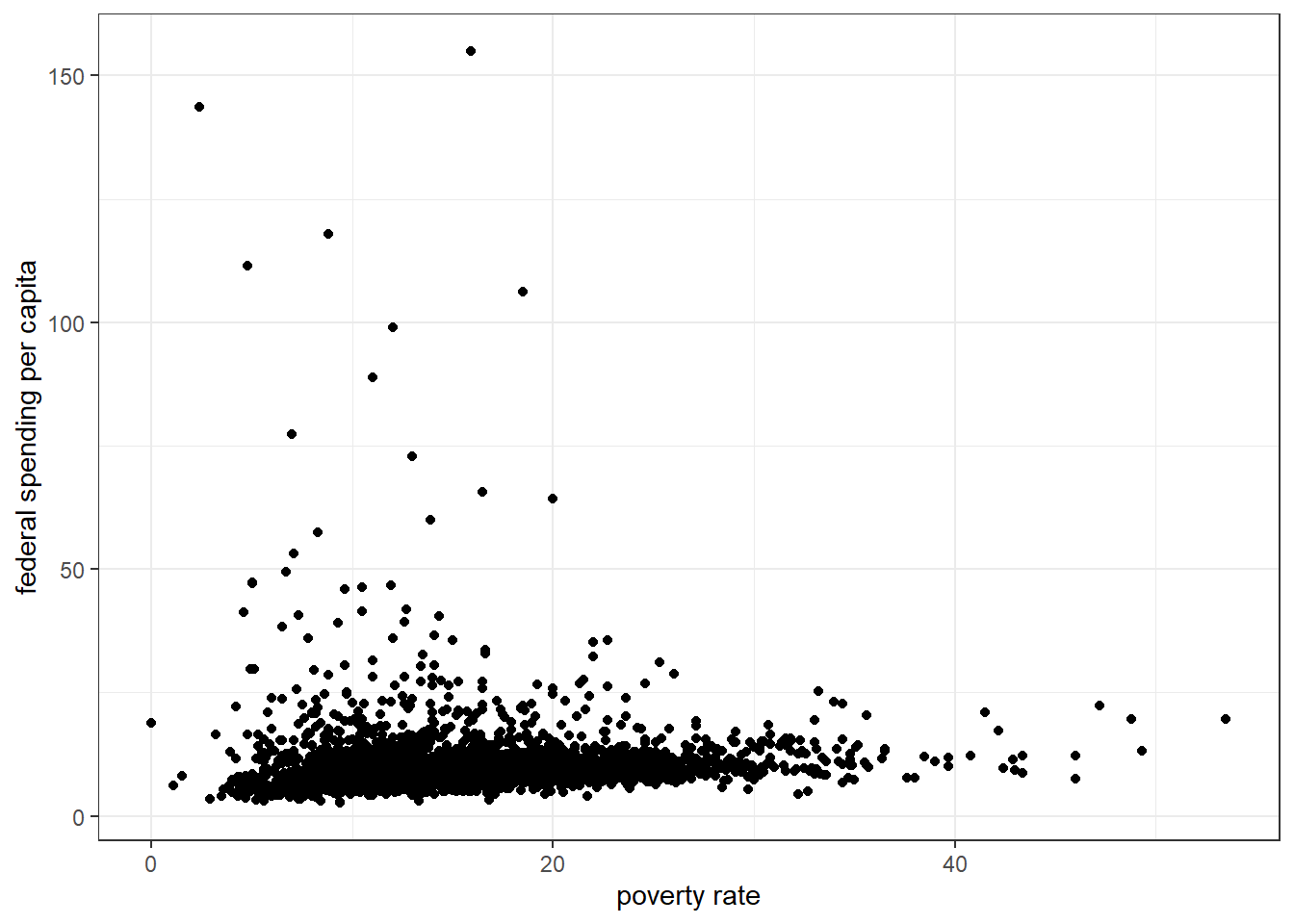## 5.6 (Q~Q) Correlation

The correlation coefficient is designated by $$r$$ for the sample correlation, and $$\rho$$ for the population correlation. The correlation is a measure of the strength and direction of a linear relationship between two variables.

The correlation ranges from +1 to -1. A correlation of +1 means that there is a perfect, positive linear relationship between the two variables. A correlation of -1 means there is a perfect, negative linear relationship between the two variables. In both cases, knowing the value of one variable, you can perfectly predict the value of the second.

### 5.6.1 Strength of the correlation

Here are rough estimates for interpreting the strengths of correlations based on the magnitude of $$r$$.

• $$|r| \geq 0.7$$: Very strong relationship
• $$0.4 \leq |r| < 0.7$$: Strong relationship
• $$0.3 \leq |r| < 0.4$$: Moderate relationship
• $$0.2 \leq |r| < 0.3:$$ Weak relationship
• $$|r| < 0.2:$$ Negligible or no relationship

### 5.6.2 Example: Federal spending per capita and poverty rate

ggplot(county, aes(x=poverty, y=fed_spend00)) +
geom_point() + ylab("federal spending per capita") +
xlab("poverty rate")cor(county$poverty, county$fed_spend00, use="complete.obs")
##  0.03484461
• There is a negligible, positive, linear relationship between poverty rate and per capita federal spending ($$r = 0.03$$).
• Let $$\rho$$ denote the true correlation between poverty rate and federal spending per capita.
• Our null hypothesis is that there is no correlation between poverty rate and federal spending ($$\rho = 0$$), and the alternative hypothesis is that they are correlated ($$\rho \neq 0$$).
• We can use the cor.test() function to analyze the evidence in favor of this alternative hypothesis.
cor.test(county$poverty, county$fed_spend00)
##
##  Pearson's product-moment correlation
##
## data:  county$poverty and county$fed_spend00
## t = 1.9444, df = 3110, p-value = 0.05194
## alternative hypothesis: true correlation is not equal to 0
## 95 percent confidence interval:
##  -0.0002922843  0.0698955658
## sample estimates:
##        cor
## 0.03484461

We conclude from this that there was a non-statistically significant, negligible correlation between poverty and federal spending ($$r = 0.03 (-0.0003, .069), p = 0.05$$).